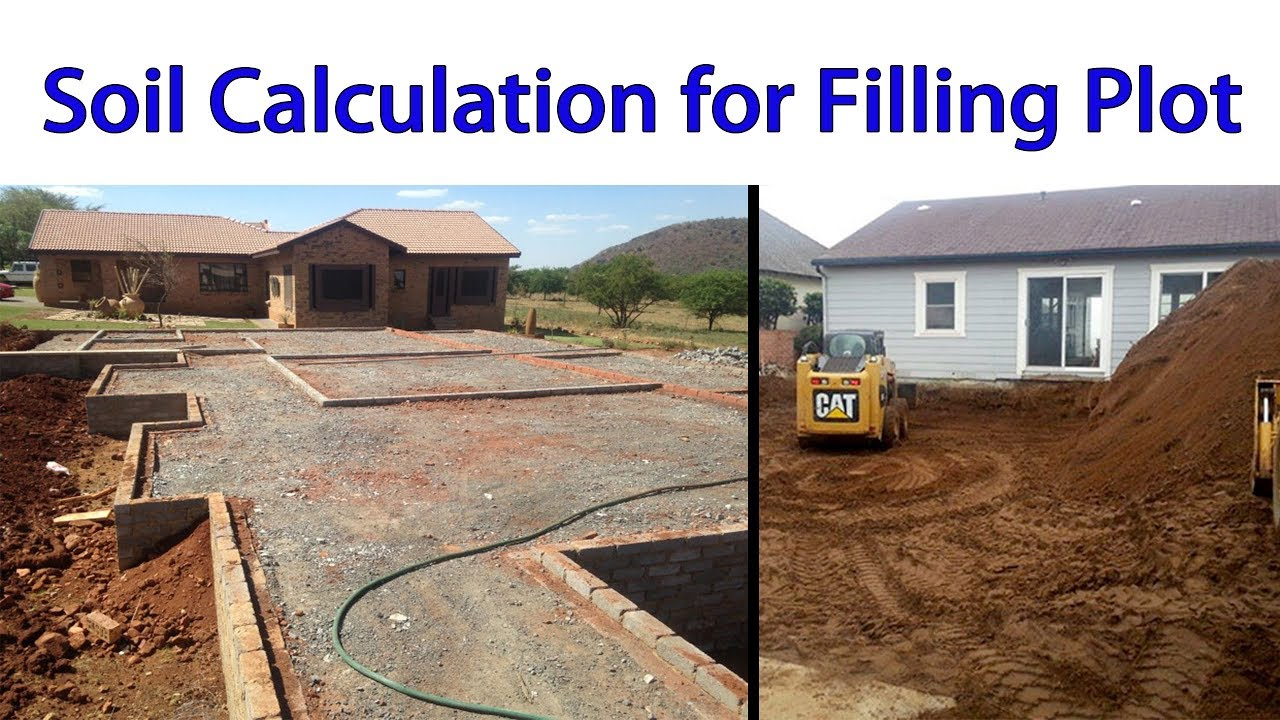Soil Calculation for Filling Plot

# Soil Calculation for Filling PlotHello friends, today In this video lecture we will discuss how to calculate materials for filling the plot. so first of all we will find the area of plot and than depth of filling , after this we will find its volume for materials requirement and than we have compaction margin that is 1.3 so we will multiply with 1.3 we can give us total materials volume.